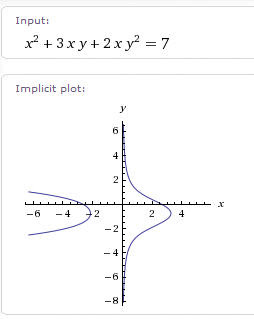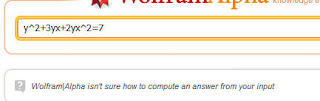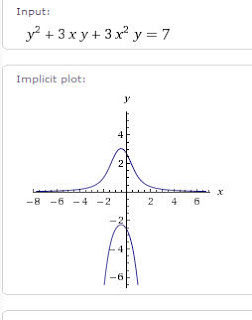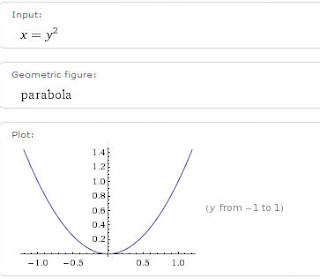## Tuesday 29 September 2009

### W' oops fram alpha?

I like Wolfram|Alpha. I use it in my classroom and even recommend it to my students as a great way to play around with mathematical ideas at home... but sometimes I get surprised at the strange things it won't do.

For instance, this morning in class.

We are working with inverse relations with my kids; trying to show that every mapping has an inverse mapping, but that doesn't mean either one of them is a function... and I showed them a complicated implicit function. I started withx^2 + 3xy + 2xy^2 = 7 just picked something off the top of my head...It was interesting, then we talked about what it would look like if we flipped it around by interchanging the x's and y's.

y^2+3yx+2yx^2=7 but???I couldn't imagine what was amiss, but retyped it as y^2 + 3xy + 3x^2 y=7Curious, I experimented...was it the order yx verses xy that confused it? tried a rectangular hyperbola

xy=1 no problem.... yx=1 this does not compute???

Ok, so what happens if I type in x=y^2... I get the typical parabola orientation you would expect with y=x^2, centered at the origin and opening up along the y? axis. Neither axis was labeled in this image, but the graph is labeled "y from -1 to 1"y^2 = x shows the right opening parabola you would expect, with both axes labeled.

One of the many idiosyncrasies of Wolfram alpha.

Joshua Zucker said...

Mathematica assumes that if you type a multi-letter string like "height" that you mean a single variable.

Same with xy and yx - a common error in Mathematica users is to type those things and forget that Mathematica thinks those are new variables rather than products.

Alpha apparently has a script that detects xy and inserts the * sign, but not the same for yx.

So, type the * signs explicitly and you'll be fine.

y^2+3y*x+2y*x^2=7 gives you the plot you want.

Pat's Blog said...

Josh, Maybe that's true, but if I write a z in place of the (yx) in each place, then I have an ellipse... and if I enter that into W_alpha, I get an ellipse, the x and y seem to be no favorites.. in fact, I just tried t^2 + r^2 = 1 and it gave me a graph of a circle... so again, I wonder why it didn't graph "something" even if it thought it was another variable.